# Discrete FP Transfer Function to FXP VI

## LabVIEW 2018 FPGA Module Help

Edition Date: March 2018
Part Number: 371599P-01
View Product InfoLabVIEW 2016 FPGA Module HelpLabVIEW 2017 FPGA Module HelpLabVIEW 2018 FPGA Module HelpLabVIEW 2019 FPGA Module HelpLabVIEW 2020 FPGA Module Help

Owning Palette: Scaling VIs

Requires: FPGA Module

Converts a transfer function system model from floating-point to fixed-point representation. Obtain this model by entering values into the FP Transfer Function Model control or by using the VIs included with the LabVIEW Control Design & Simulation Module.

You then can implement this model on an FPGA by using the Discrete Transfer Function Direct VI.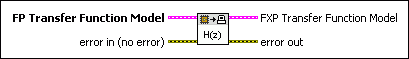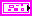FP Transfer Function Model specifies the floating-point transfer function controller model this VI converts to fixed-point representation. The model you wire to this terminal must meet the following requirements: The model must be in transfer function form. If the model is in zero-pole-gain or state-space form, use the Model Conversion VIs to convert the model to transfer function form. These VIs are available with the LabVIEW Control Design & Simulation Module. The order of the denominator must be less than or equal to eight. The transfer function must be proper; that is, the order of the denominator must be greater than or equal to the order of the numerator. The model must be in the simulation data type. If you created the model with the LabVIEW Control Design & Simulation Module, you must use the CD Convert Control Design to Simulation VI to convert the model to the simulation data type. The model must be discrete. If the model is continuous, use the CD Convert Continuous to Discrete VI, available with the Control & Simulation Module, to discretize the model. The sampling time of the discrete model must match the execution rate of the FPGA. To calculate the proper sampling time and FPGA execution rate, take the following factors into account: The rate at which the controller accepts input u(k) from a sensor The rate at which the actuator accepts input y(k) from the controller Ideally, these rates will be equal. In this situation, set the sampling time of the model and the FPGA execution rate to this rate. For example, if the controller accepts input at 1 kHz and the actuator accepts the controller output at 1 kHz, set the sampling time of the model to 1/1,000 or 0.001 s. Set the FPGA execution rate to 1 kHz. If these two rates are different, set the sampling time and FPGA execution rate to the faster of these two rates. For example, if the controller accepts input at 1 kHz but the actuator accepts the controller output at 2 kHz, set the sampling time to 1/2,000 or 0.0005 s. Set the FPGA execution rate to 2 kHz.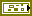error in describes error conditions that occur before this node runs. This input provides standard error in functionality.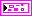FXP Transfer Function Model returns the FP Transfer Function Model in fixed-point representation. Wire this output to the FXP Transfer Function Model input of the Discrete Transfer Function Direct VI.Each element of FXP Transfer Function Model has a data type of <+/–, 32, 12>. You can change this data type, but before doing so you must right-click this indicator and select Disconnect from Type Def. from the shortcut menu.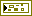error out contains error information. This output provides standard error out functionality.